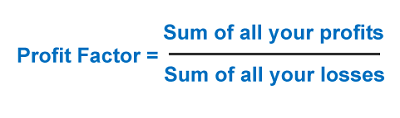# Profit factor – Definition and CalculationThe profit factor is one of the most popular performance metrics used in trading.

Its calculation is very simple: in the formula, the total amount earned in the positive trades is divided by the total lost in the trades with losses.

## Formula for calculating the profit factor

This is the simplest way to calculate this indicator:

Profit Factor = Gross Profit / Gross Loss

Profit Factor = Sum of profits from winning trades/Sum of losses from losing trades

If you want to complicate the issue a bit more, we can also calculate the profit factor using the percentage of winning trades and the average profit and loss. This way the formula would look like this:

Profit Factor = (Average gain of winning trades x Percentage of winning trades)/(1-Percentage of winning trades) x Average loss of trades

Anyway, with both formulas, we get the same result.

## What is the optimal value of this ratio?

If the trading strategy we are analyzing is profitable, obviously the gross profit will be greater than the gross loss, resulting in a profit factor greater than 1.

That is why we will always look for strategies with ratios clearly above 1 and reject strategies with lower profit factors.

## What is the ideal profit factor?

As a general rule, we will seek to make the profit factor as high as possible. How much? Preferably greater than 2 (although around 1.6 we can say that the strategy can be worthwhile) and we can say that a profit factor much greater than 3 is something exceptional.

But you have to keep in mind that the profit factor, like all metrics to evaluate trading systems, is calculated over a given period. As the calculation period is extended or varied, the ratio changes.

## Benefits of the profit factor as a performance analysis metric

In addition to simplicity in its calculation, the main advantage of the profit factor is that this ratio clearly indicates how much we earn for each dollar or euro we lose.

A profit factor equal to 1 tells us that for every dollar we lose we will win 1. A profit factor equal to 2.5 tells us that this system has earned two and a half dollars for every dollar it has lost. In this way, with the profit factor, we can have a clear vision of the performance of the trading system.

## Disadvantages of this indicator

What is the problem of using the profit factor as the sole performance measure of our trading strategies?

That this ratio tells us nothing about the trades distribution.

Having a profit factor greater than 1 does not mean being a consistent trader.

So we can have a positive profit factor due to a single trade with a great benefit (perhaps due only to chance) even if the rest of our trades are closed in losses.

That is why, although the profit factor gives us a clear vision of the trading system’s performance, it is always necessary to see the global picture and put the result of the ratio in context:

• How many transactions does the system have?
• Does the maximum drawdown exceed our risk limit?
• Is it a system consistent in its results or does it have a lot of dispersion?
• What is the ratio of winning trades?
• Is the Sharpe ratio too low?
• What is the average profit per trade?
• Etc.

To answer this, the trader must use a list with the main ratios to evaluate a trading system. The different metrics are complementary and together they help us to have a better perspective of a system before making decisions.